# Extraction of Dynamical Equations from Chaotic Data

G. Rowlands and J. C. Sprott
Department of Physics, University of Warwick, Coventry CV47AL, England
Department of Physics, University of Wisconsin, Madison, WI 53706, USA
Revised manuscript received 29 January 1992
Accepted 7 February 1992

### ABSTRACT

A method is described for extracting from a chaotic time series a system of equations whose solution reproduces the general features of the original data even when these are contaminated with noise. The equations facilitate calculation of fractal dimension, Lyapunov exponents and short-term predictions. The method is applied to data derived from numerical solutions of the logistic equation, the Henon equations with added noise, the Lorenz equations and the Rossler equations.

Ref: G. Rowlands and J. C. Sprott, Physica D 58, 251-259 (1992)

The complete paper is available in PDF format.

Fig. 1. Phase-space plots of the logistic equation (a) from original data and (b) after singular value decomposition.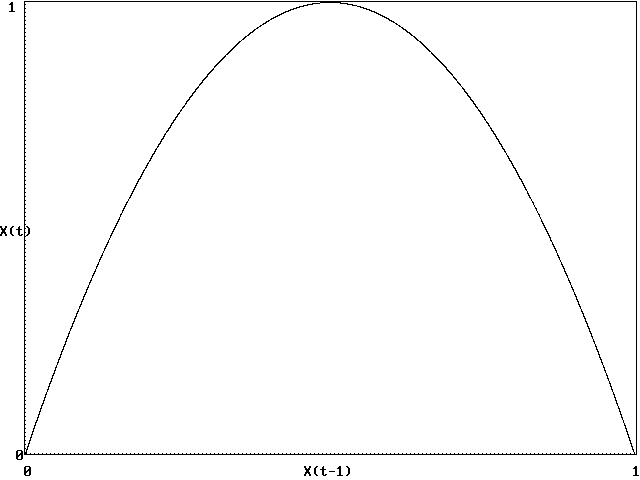(a)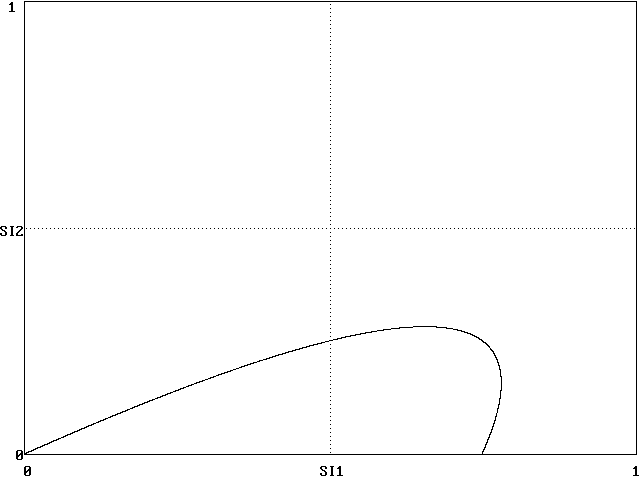(b)

Fig. 2. Phase-space plots of the Henon map (a) from original data and (b) after singular value decomposition.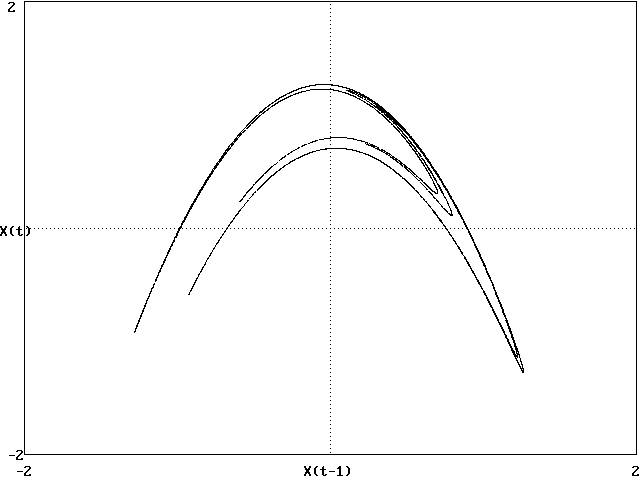(a)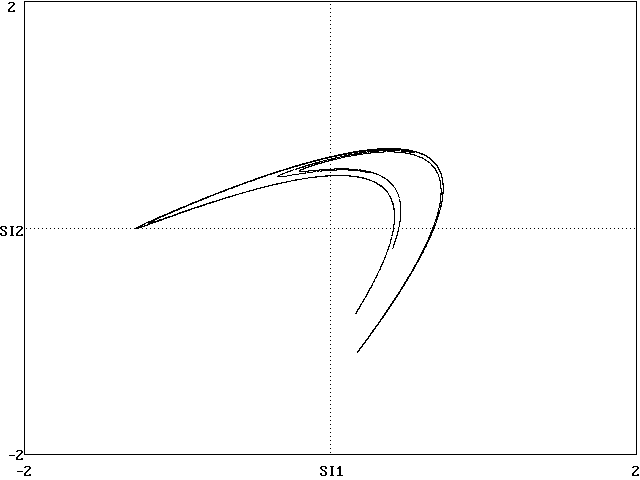(b)

Fig. 3. Phase-space plots of the Henon map (a) with noise added and (b) from a solution of the model equations fit to the noisy data. A comparison of (b) with fig. 2a shows that the method has completely removed the noise and restored the original.(a)(b)

Fig. 4. Three-dimensional phase-space plots of the Lorenz attractor showing that the topology of the attractor is preserved. (a) Original input data, (b) result of singular value decomposition, and (c) solution of the model equations.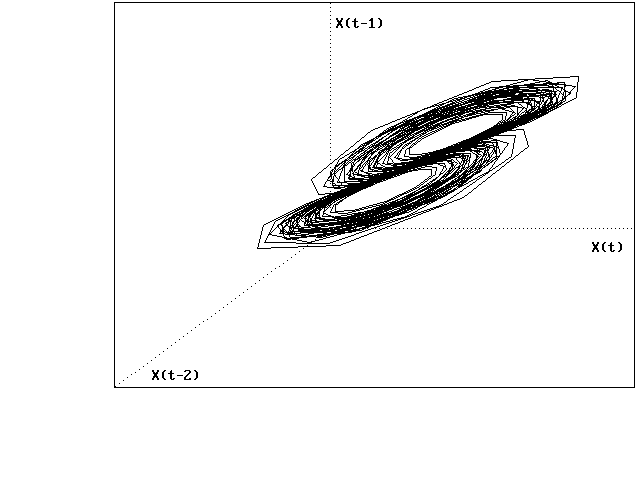(a)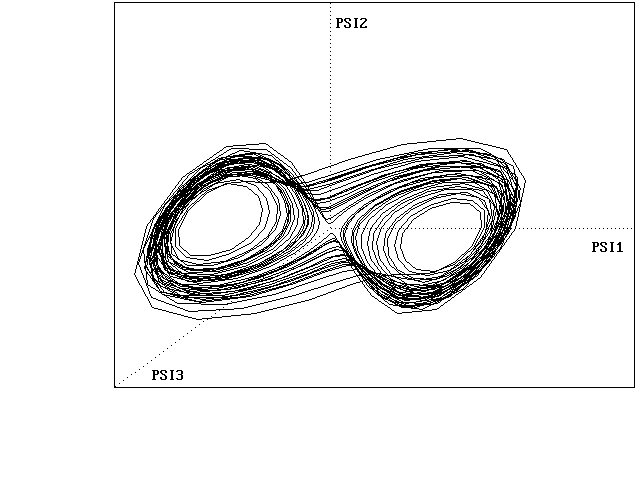(b)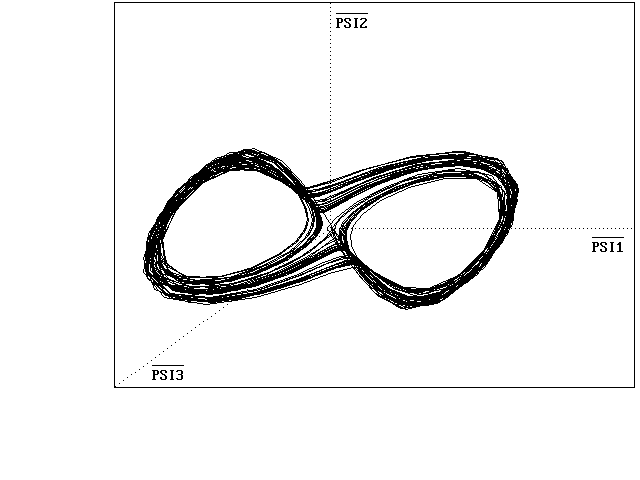(c)

Fig. 5. Three-dimensional phase-space plots of the Rossler attractor showing that the topology of the attractor is preserved. (a) Original input data, (b) result of singular value decomposition, and (c) solution of the model equations.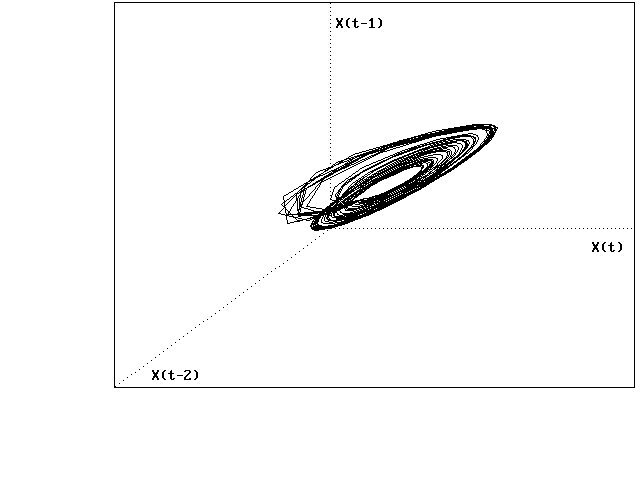(a)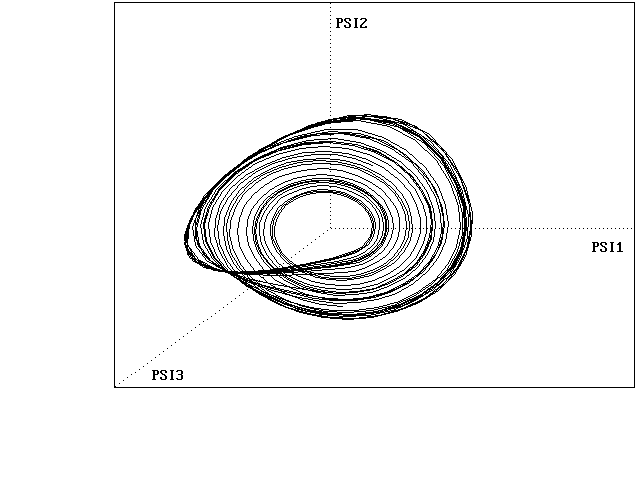(b)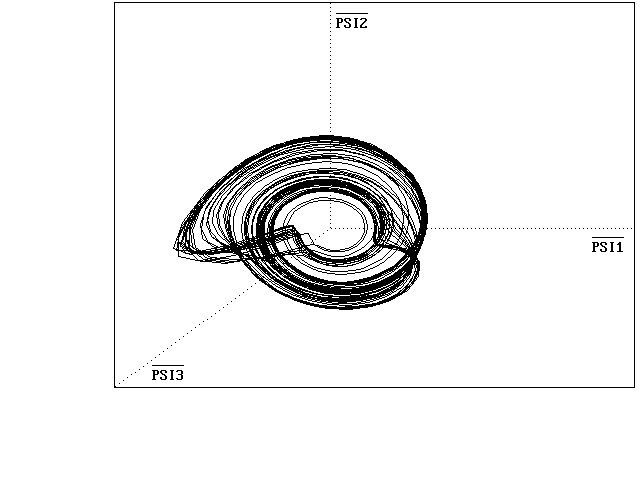(c)

Fig. 6. (a) Variation of the nine largest coefficients of the model equations with the parameter r in the Lorenz equations, (b) along with a least squares fit of each coefficient to a cubic polynomial in r.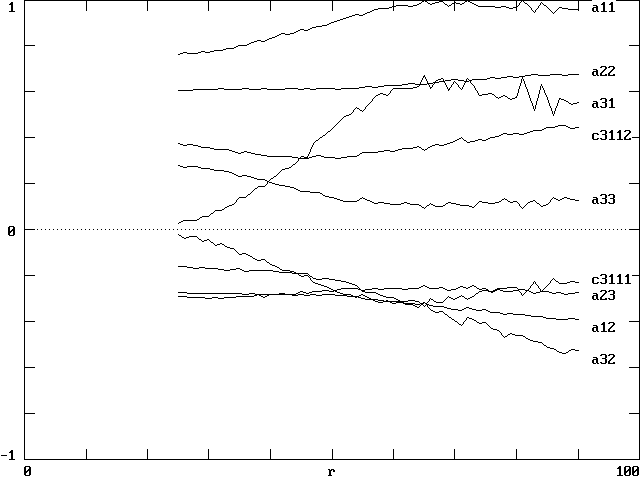(a)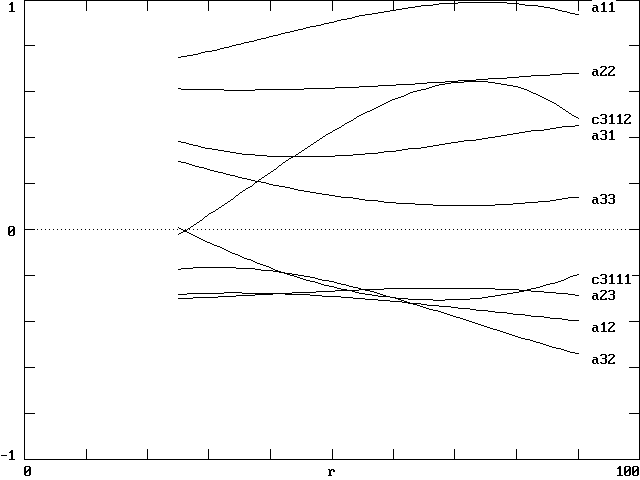(b)

Fig. 7. Phase-space portraits for the Lorenz attractor with r=57 (a) obtained directly from singular value decomposition, and (b) resulting from solution of the model equations with coefficients calculated from least squares fits to cubic polynomials in r.